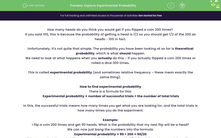# Explore Experimental Probability

In this worksheet, students will practise finding experimental probability and compare it to theoretical probability.Key stage:  KS 4

Year:  GCSE

GCSE Subjects:   Maths

GCSE Boards:   AQA, Eduqas, Pearson Edexcel, OCR,

Curriculum topic:   Probability

Curriculum subtopic:   Probability Basic Probability and Experiments

Popular topics:   Probability worksheets

Difficulty level:#### Worksheet Overview

How many heads do you think you would get if you flipped a coin 200 times?

If you said 100, this is because the probability of getting a head is 1/2 so you should get 1/2 of the 200 as heads - 100 in fact.

Unfortunately, it's not quite that simple. The probability you have been looking at so far is theoretical probability, which is what should happen.

We need to look at what happens when you actually do this - if you actually flipped a coin 200 times or rolled a dice 300 times.

This is called experimental probability (and sometimes relative frequency - these mean exactly the same thing).

How to find experimental probability

There is a formula for this:

Experimental probability = number of successful trials ÷ the number of total trials

In this, the successful trials means how many times you get what you are looking for, and the total trials is how many times you do the experiment.

Example:

I flip a coin 200 times and get 90 heads. What is the probability that my next flip will be a head?

We can now just bang the numbers into the formula:

Experimental probability = 90 ÷ 200 = 90/20

This means that the probability of my next flip being a head is 9/20 (Don't forget to cancel down if you can)

The link between theoretical and experimental probability

We now know that theoretical probability is what should happen and experimental probability is what does happen.

The thing you need to know here is that the more times you do an experiment, the closer the experimental probability will get to the theoretical probability.

The way to test your understanding of this is through asking questions where one person does an experiment more times than another. Which one will be more accurate? Always the one who has done more.

Let's have a go at some questions then.

### What is EdPlace?

We're your National Curriculum aligned online education content provider helping each child succeed in English, maths and science from year 1 to GCSE. With an EdPlace account you’ll be able to track and measure progress, helping each child achieve their best. We build confidence and attainment by personalising each child’s learning at a level that suits them.

Get started